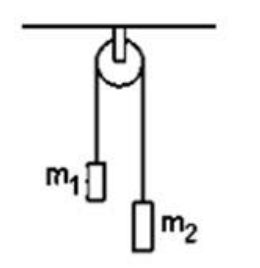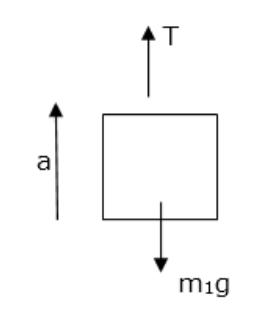Deepak Scored 45->99%ile with Bounce Back Crack Course. You can do it too!

# Solve the following :

Question:

In a simple Atwood machine, two unequal masses $\mathrm{m} 1$, and $\mathrm{m} 2$ are connected by a string going over a clamped light smooth pulley. In a typical arrangement $\mathrm{m} 1=300 \mathrm{~g}$ and $\mathrm{m} 2=600 \mathrm{~g}$. The system is released from rest. (a) Find the distance travelled by the first block in the first two seconds. (b) Find the tension in the string. (c) Find the force exerted by the clamp on the pulley.Solution:$\mathrm{T}-\mathrm{m}_{1} \mathrm{~g}=\mathrm{m}_{1 \mathrm{a}}$

$m_{1} g+m_{2} a=m_{2}(g-a)$

$\left(m_{1}+m_{2}\right) a=\left(m_{2}-m_{1}\right) g$

$a=\frac{0.6-0.3}{0.6+0.3} \times 9.8=3.266 \mathrm{~m} / \mathrm{s}^{2}$

$a=\frac{0.6-0.3}{0.6+0.3} \times 9.8=3.266 \mathrm{~m} / \mathrm{s}^{2}$

a) use $\mathrm{x}_{-\mathrm{x}_{0}=} u t+\frac{1}{2} a t^{2}$

$=\frac{1}{2}(3.266) \times 2^{2}=6.5 \mathrm{~m} \quad[u=0]$

b) $\mathrm{T}=\mathrm{m}_{1}(\mathrm{~g}+\mathrm{a})=\mathrm{m}_{1}(9.8+3.266)$

$=0.3(13.06)$

$=3.918 \mathrm{~N}$

c) $F=2 T=2 \times 3.918=7.8 \mathrm{~N}$